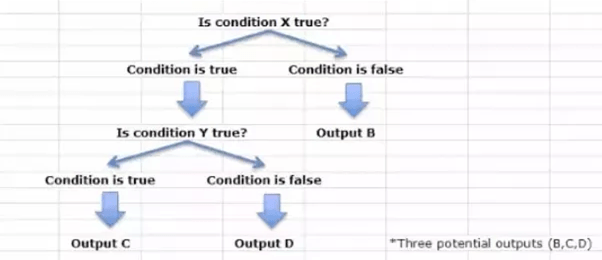# IF Statement with Three Outcomes

IF statement is used to test whether a certain condition is met. Sometimes you can have more than one condition to test thus creating an If statement within another if statement (nested if statement). This enables you to come up with if statement with many possible outcomes as you want.

The excel If () function is used when evaluating a condition with two outcomes. e.g. (evaluate if sales are greater than 1000 and give different values for each outcome). The function is commonly used to evaluate whether a condition is true or false.

It returns one outcome if the logical; test is TRUE and another outcome if the condition is FALSE.

The syntax for two outcomes

IF(logical_test, [value_if_True], [value_if_False])

There are situations where you have to work with more than two outcomes. In such a situation, you're required to create Multiple IF statements or Nested If Statements.

How to use multiple if statements with more than two outcomes

This multiple if statement works by replacing one of the True/False calculations with another if function. You create and if function within another if function.Syntax

=IF (CONDITION X, OUTPUT B, IF (CONDITION Y, OUTPUT C, OUTPUT D)))

Example: Commission for sales based on monthly sales

Open your excel sheet and enter the following detailsFrom the above table, create an if statement with the following conditions

 Sales Commission Less than \$400 7% Sales between \$400 and \$800 12.5% Sales above \$800 16%

Use nested if to calculate the commission for Shelly.

Perform a logical test on sales: Is the Sales figure less than \$400? If TRUE, then calculate commission. If FALSE, then is the sales less than \$800? If TRUE then calculate the commission and if False, then calculate the commission.

Enter the following formula in cell C2

=IF(B2<400,B2*7%,IF(B2<800, B2*12.5%,B2*16%))Press enter key to calculate the commission for Shelly.

Use the Autofill feature to copy the formula to the rest of the sales team.In the above example, the If statement checks if cell B2 is greater than less than \$400. If that is true, it calculates the commission with 7% and stops calculating. If cell B2 had more than \$400 and sales of less than \$800, the commission will be calculated at 12.5% otherwise if the sales figure is greater than \$800 commission will be calculated at a rate of 16%.